• +91 9971497814
• info@interviewmaterial.com

# RD Chapter 1- Real Numbers Ex-1.1 Interview Questions Answers

### Related Subjects

Question 1 : If a and b are two odd positive integers such that a > b, then prove that one of the two numbers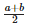and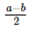is odd and the other is even.

a and b are two odd numbers such that a > b
Let a = 2n + 1, then b = 2n + 3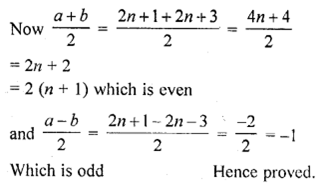Question 2 : Prove that the product of two consecutive positive integers is divisible by 2.

Let n and n + 1 are two consecutive positive integer
We know that n is of the form n = 2q and n + 1 = 2q + 1
n (n + 1) = 2q (2q + 1) = 2 (2q2 + q)
Which is divisible by 2
If n = 2q + 1, then
n (n + 1) = (2q + 1) (2q + 2)
= (2q + 1) x 2(q + 1)
= 2(2q + 1)(q + 1)
Which is also divisible by 2
Hence the product of two consecutive positive integers is divisible by 2

Question 3 : Prove that the product of three consecutive positive integer is divisible by 6.

Answer 3 : Let n be the positive any integer Then
n(n + 1) (n + 2) = (n2 + n) (n + 2)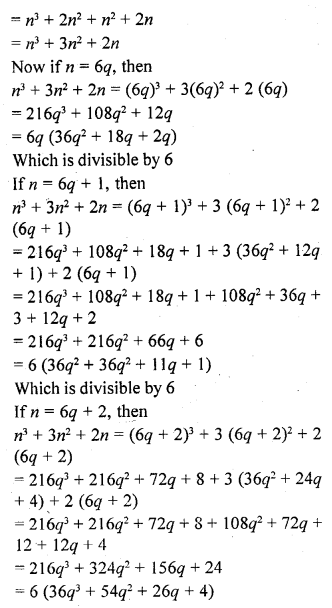Which is also divisible by 6
Hence the product of three consecutive positive integers is divisible by 6

Question 4 : For any positive integer n, prove that n3 – n is divisible by 6.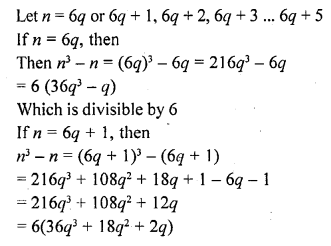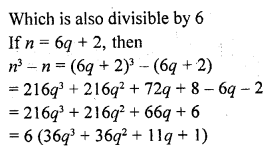Which is divisible by 6
Hence we can similarly, prove that n2 –n is divisible by 6 for any positive integer n.
Hence proved.

Question 5 : Prove that if a positive integer is of the form 6q + 5, then it is of the form 3q + 2 for some integer q, but not conversely.

Let n = 6q + 5, where q is a positive integer
We know that any positive integer is of the form 3k or 3k + 1 or 3k + 2, 1
q = 3k or 3k + 1 or 3k + 2
If q = 3k, then n = 6q + 5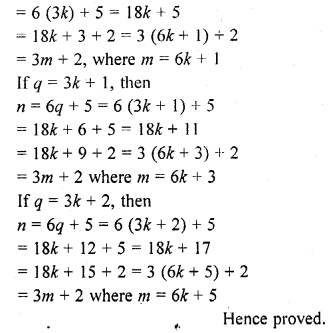Question 6 : Prove that the square of any positive integer of the form 5q + 1 is of the same form.

Let a be any positive integer
Then a = 5m + 1
a2 = (5m + 1 )2 = 25m2 +10m + 1
= 5 (5m2 + 2m) + 1
= 5q + 1 where q = 5m2 + 2m
Which is of the same form as given
Hence proved.

Question 7 : Prove that the square of any positive, integer is of the form 3m or, 3m + 1 but not of the form 3m + 2.

Let a be any positive integer
Let it be in the form of 3m or 3m + 1
Let a = 3q, then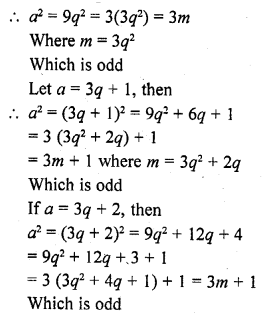Hence proved.

Question 8 : Prove that the square of any positive integer is of the form 4q or 4q + 1 for some integer q.

Let a be the positive integer and
Let a = 4m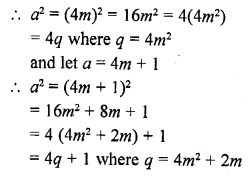Hence proved.

Question 9 : Prove that the square of any positive integer is of the form 5q, 5q + 1, 5q + 4 for some integer q.

Let a be the positive integer, and
Let a = 5m, then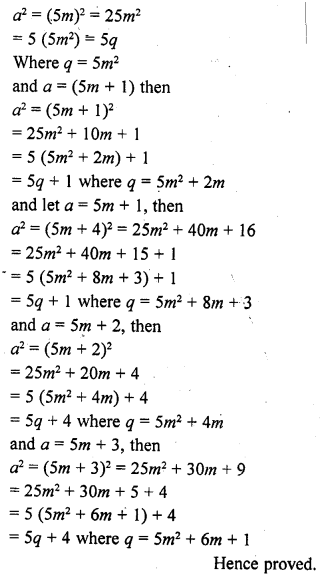Question 10 : Show that the square of an odd positive integer is of the form 8q + 1, for some integer q.

Let n is any positive odd integer
Let n = 4p + 1, then
(4p + 1)2 = 16p2 + 8p + 1
n2 = 8p (2p + 1) + 1
= 8q + 1 where q = p(2p + 1)
Hence proved.

Todays Deals### RD Chapter 1- Real Numbers Ex-1.1 Contributorskrishan

Name:
Email:

# Latest News# 9000 interview questions in different categories